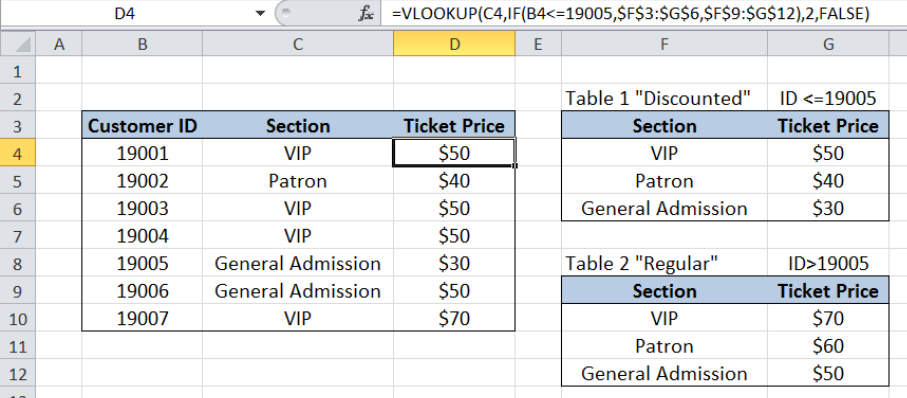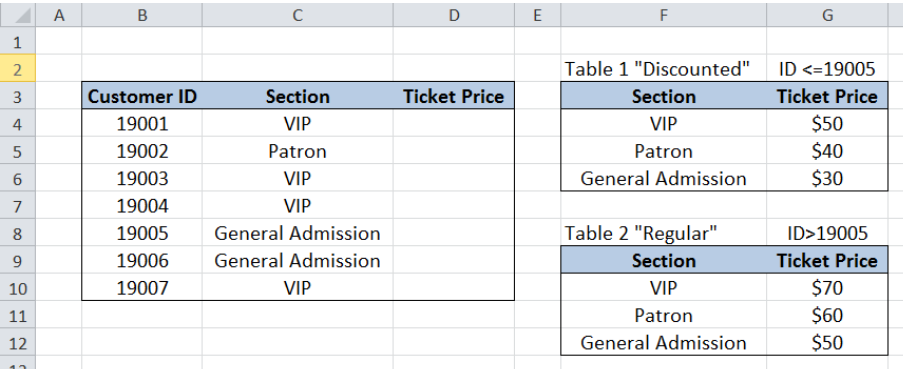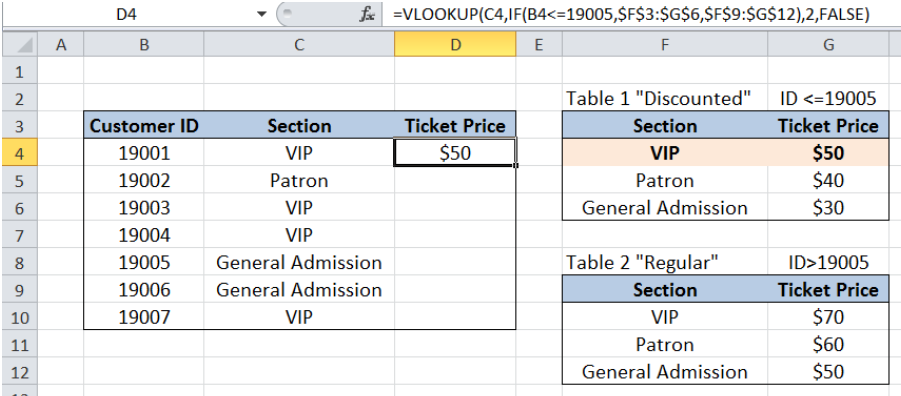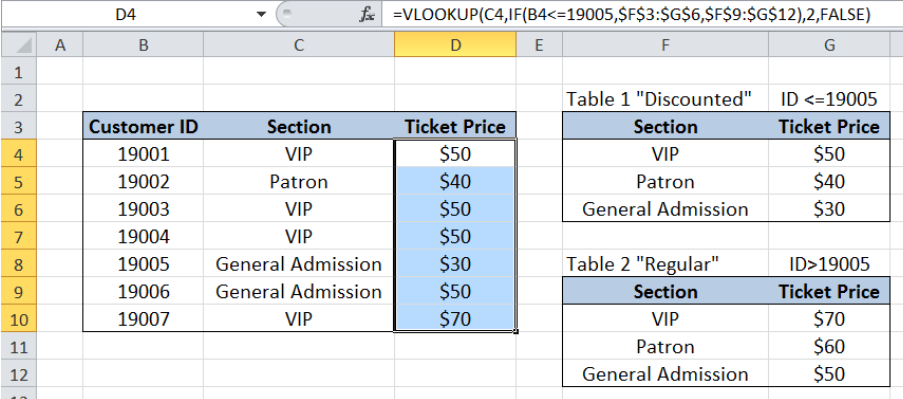Get instant live expert help with Excel or Google Sheets“My Excelchat expert helped me in less than 20 minutes, saving me what would have been 5 hours of work!”

#### Post your problem and you'll get expert help in seconds

Your message must be at least 40 characters
Our professional experts are available now. Your privacy is guaranteed.

# VLOOKUP with 2 lookup tables

While working with Excel, we are able to lookup a value and retrieve data from a data set using the VLOOKUP function.  However, when we need to work with two lookup tables, we retrieve data from the appropriate table by using the VLOOKUP and IF functions. This step by step tutorial will assist all levels of Excel users in using VLOOKUP with two lookup tables.Figure 1. Final result: VLOOKUP with two lookup tables

Final formula:  `=VLOOKUP(C4,IF(B4<=19005,\$F\$3:\$G\$6,\$F\$9:\$G\$12),2,FALSE)`

## Syntax of the VLOOKUP function

VLOOKUP is used when we want to look up a value and retrieve data from a given data set.

`=VLOOKUP(lookup_value, table_array, col_index_num, [range_lookup])`

The parameters of the VLOOKUP function are:

• lookup_value – the value that we want to search and find in the table_array
• table_array – the range of cells in the source table containing the data we want to retrieve
• col_index_num the column number in the table_array corresponding to the information we want to retrieve, relative to the lookup_value
• [range_lookup] – optional; value is either TRUE or FALSE
• if TRUE or omitted, VLOOKUP returns either an exact or approximate match
• if FALSE, VLOOKUP will only find an exact match

## Syntax of the IF function

IF function evaluates a given logical test and returns a TRUE or a FALSE

`=IF(logical_test, [value_if_true], [value_if_false])`

• The arguments “value_if_true” and “value_if_false” are optional.  If left blank, the function will return TRUE if the logical test is met, and FALSE if otherwise.

## Setting up Our Data

Our table contains a list of Customer ID (column B), Section (column C) and Ticket Price (column D).  We want to determine the ticket price based on the section that the customer chooses. However, there is a discount given to the first five customers with customer ID from 19001 to 19005.

In columns F and G, we have two tables showing the prices per section.  Table 1 F3:G6 contains the discounted prices while Table 2 F9:G12 contains the regular prices.Figure 2. Sample data for VLOOKUP with two lookup tables

We want to populate column D with the corresponding ticket price, based on the conditions given.

## Lookup ticket price with 2 lookup tables

We want to determine the ticket price based on Customer ID and section.  When working with two lookup tables, we need to incorporate the IF function within the VLOOKUP formula.

Our formula looks like this:

`=VLOOKUP(C4,IF(B4<=19005,\$F\$3:\$G\$6,\$F\$9:\$G\$12),2,FALSE)`

Our lookup_value is cell C4 which is the section.  Our table_array is the IF function IF(B4<=19005,\$F\$3:\$G\$6,\$F\$9:\$G\$12).  It tests if the Customer ID (B4) is less than or equal to 19005.   If the test returns TRUE, the table array is F3:G6; otherwise, the table array becomes F9:G12.

In order to determine the ticket price using VLOOKUP with two lookup tables, we follow these steps:Figure 3. Entering the formula to lookup ticket price

Step 1.  Select cell D4

Step 2.  Enter the formula: `=VLOOKUP(C4,IF(B4<=19005,\$F\$3:\$G\$6,\$F\$9:\$G\$12),2,FALSE)`

Step 3:  Press ENTER

Step 4:  Copy the formula in cell D4 to cells D5:D10 by clicking the “+” icon at the bottom-right corner of cell D4 and dragging it down

The dollar signs “\$” in the formula fix the cells so that we can easily copy and paste the formula to other cells.

“Ticket Price” is in the second column of the table array so col_index_num is 2.  Range_lookup is FALSE because we want to find the exact match.

The final result in cell D4 is \$50, which is the discounted price for a VIP ticket, since the Customer ID is less than 19005.

Copying the formula to the succeeding cells populates column D with the ticket prices based on Customer ID and section.Figure 4. Output: VLOOKUP with two lookup tables

Note:  When VLOOKUP doesn’t find a match to our lookup value, the function returns the value #N/A.

Most of the time, the problem you will need to solve will be more complex than a simple application of a formula or function. If you want to save hours of research and frustration, try our live Excelchat service! Our Excel Experts are available 24/7 to answer any Excel question you may have. We guarantee a connection within 30 seconds and a customized solution within 20 minutes.

### Did this post not answer your question? Get a solution from connecting with the expert.Another blog reader asked this question today on Excelchat:## Subscribe to Excelchat.coAnother blog reader asked this question today on Excelchat: